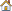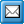﻿ Customizing Element Symbols in QPR Modeling Client Using XML
QPR Knowledge Base 2017.1

Customizing Element Symbols in QPR Modeling Client Using XMLDeveloper's Guide:

Customizing Element Symbols in QPR Modeling Client Using XMLCustomizing Element Symbols in QPR Modeling Client Using XMLThe shape of an element symbol used in QPR Modeling Client can be defined by using an XML file. The shape consists of paths that define the symbol, and they also contain a list of connection points where connectors can be connected to.

The following tags are used to define the element symbol in an XML file:

Shape: This is the first tag in the XML file.

Path: Definitions inside the Path define how the element symbol is drawn. The following tags can be used inside a Path:

oPen: Used for drawing a path. If the pen is not defined or it uses the "default" value, the line style defined in the graphical properties of the symbol is used.  The Pen has the following attributes:

Style="default": line is drawn with the style taken from the graphical properties of the symbol, "solid": draws a solid line, or "custom":  draws a custom pattern line defined in the same way as the Custom Dash Pattern in the graphical properties of the symbol.

Width="default": the width defined in the graphical properties of the symbol is used or "<value>": the width in pixels.

Color="default": the color defined in the graphical properties of the symbol is used or "<value>": an RGB value.

Transparency="default": the transparency defined in the graphical properties of the symbol is used or "<value>": a percentage value from 0 to 100.

oBrush: Used for filling the path. If the brush is not defined or uses the "default" value, the one defined in the graphical properties of the symbol is used. The Brush has the following attributes:

Style="default": the fill style defined in the graphical properties of the symbol is used, "solid": a solid fill is used "rectgradient": a rectangle gradient is used, "linegradient": a line gradient it used, or "hatch": a hatch with a defined hatch pattern index is used.

Color="default": the color defined in the graphical properties of the symbol is used or "<value>": a single RGB value for solid style, or two RGB values separated by a comma for the gradient and hatch styles.

oShadow: Used for defining a shadow for the element. If a shadow is defined in the XML file used, it is drawn even when it is not defined to be drawn in the symbol type settings. If a shadow is defined to be drawn in the symbol type settings, it is drawn even when it is not defined in the XML file used. If a shadow is not defined, the one defined in the the graphical properties of the symbol is used. The Shadow has the following attributes:

Color="default": the color defined in the graphical properties of the symbol is used or "<value>": an RGB value.

Transparency="default": the transparency defined in the graphical properties of the symbol is used or "<value>": a percentage value from 0 to 100.

Size="default": the shadow defined in the graphical properties of the symbol is used or "<value>": percentage value from 0 to 200.

Blur="default": the blur defined in the graphical properties of the symbol is used or "<value>": a value between 0 and 100.

Angle="default": the angle defined in the graphical properties of the symbol is used or "<value>": a value between 0 and 359.

Distance="default": the distance defined in the graphical properties of the symbol is used or "<value>": a value between 0 and 200.

oHighlight: Defines if the path is used for highlighting the element, for example when connecting a flow to it. Multiple paths in the element can be highlighted.

oPoint: Defines a point in the path. The point is used as a starting point for lines and arcs. The point can be defined as a fixed coordinate or its values can be calculated from the width and the height of the symbol using addition, subtraction, multiplication, division (+ - * /), and round function operations, as well as parenthesis to define the order of the operations. In addition to the width and height of the symbol, gridx and gridy variables can be used to define the values from the diagram options even if the grid is not used. Also percentage values are supported. Percentage values will use either the width or the height of the symbol. The Point has the following attributes:

x="<value>": defines the X coordinate using either width or gridx as the defined variable.

y="<value>": defines the Y coordinate using either height or gridy as the defined variable.

For example:

a point two thirds of symbol's width from left edge, 16 pixels from the top of the symbol: <Point x="width*2/3" y="16" />.

a point 16 pixels + half of the rest of symbol's height from the top of the symbol, on symbol's right edge <point x="width" y="16+(height-16)/2" />

a point 30% of the symbol's width from the left edge 22% from the top of the symbol: <Point x="30%" y="22%" /> would be the same as <Point x="width*30/100" y="height*22/100" />.

oLineto: Defines a straight line from the previous point to the point defined in the line. The Lineto has the following attributes:

x="<value>": defines the X coordinate of the line destination.

y="<value>": defines the Y coordinate of the line destination.

relative="true": makes the X and Y coordinates given relative to the previous point instead of the coordinates being absolute.

For example:

a line that is drawn to the right from the current point ending to a point 10 pixels away from the right border of the symbol: <Lineto x="width-10" relative="true">

oArc: Defines an arc contained in a rectangle defined by the attributes. The Arc has the following attributes:

x1="<value>": the first coordinate in the rectangle.

x2="<value>": the second coordinate in the rectangle.

y1="<value>": the third coordinate in the rectangle.

y2="<value>": the fourth coordinate in the rectangle.

startangle="<value>": defines where the arc starts, 0 being straight right from the center, 90 straight down etc.

sweepangle="<value>": defines the length of the arc section in degrees, i.e. 180 being a semicircle.

direction="clockwise": the arc is drawn clockwise or "anticlockwise": the arc is drawn anticlockwise.

oClosefigure: Closes the path. The path is also closed when a line is drawn to the starting point of the path.

To preserve the aspect ratio for a path, it's enough to refer either to the symbol width or the symbol height (but not both) in all the coordinates of its parts.

Connectionpoints: Points inside the Connectionpoints tag define the points where connectors can connect to the symbol. A Point inside the Connectionpoints tag has the following attributes:

ox="<value>": the x position of the connection point.

oy="<value>": the y position of the connection point.

odirections="<list>": a comma-separated list of directions from where an elbow style connector can connect to the connection point. Possible directions are right, left, top, and bottom.

osnaptoxgrid="true": defines the connectionpoint to snap to the x grid.

osnaptoygrid="true": defines the connectionpoint to snap to the y grid.

For example:

<Connectionpoints>

<Point x="width*2/3" y=o directions="right,top" SnapToXGrid="true" />

</Connectionpoints>

A Connectionpoint (note that this is written without the 's')  definition can also be done in the Point, Lineto, and Arc definitions. The definition is otherwise similar to what is described above, but the x and y coordinates are replaced with Position as follows:

oFor Point, the Position is omitted as the connectionpoint is always the same as the point itself.

oFor Lineto, the Position is a percentage or a fixed pixel value from the starting point of the line. If the position is outside the line, it is set to the end of that line.

oFor Arc, the Position is a percentage or a fixed degree angle value from the start angle of the arc. If the position is outside the arc, it is set to the end of that arc.

For example:

<Point x="0" y="0" />

<Lineto x="width" y="0">

<Connectionpoint position="50%" SnapToXGrid="true" />

</Lineto>

In this case, if the element is on the diagram at position Left=40, Top=25, its width=150 and height=100, the connection point would be at the half point of the line x=(Left + width * 50%) = (40 + 150 * 50%) = 115, y=(Top) = 25. The SnapToXGrid option snaps the x coordinate to the closest grid point (assuming the grid size = 10 pixels) -> x=120, y=25. In any case, the connection point will not be moved beyond the line start/end points.

If an XML defined shape has connectionpoints defined, then only those connection points are used. If not, only the default connection points of the background shape are used.

Examples of an XML Shape Definition File:

Example 1:

<?xml version="1.0"?>

<Shape>

<Path>

<Pen style="default" width="3" color="default" />

<Brush style="solid" color="\$80000000" />

<Shadow color="default" transparency="50" size="100" blur="2" angle="45" distance="10" />

<Point x="0" y="0" />

<Lineto x="100%" relative="true" />

<Lineto y="100%" relative="true" />

<Lineto x="-100%" relative="true" />

<Closefigure />

<Highlight />

</Path>

<Path>

<Pen style="custom:5 2 10 4" width="default" color="\$80FF00FF" />

<Brush style="default" color="\$80FF0000" />

<Arc x1="gridx" y1="gridy" x2="width-gridx" y2="height-gridy" startangle="0" sweepangle="360" direction="anticlockwise" />

</Path>

<Connectionpoints>

<Point x="width/2" y="0" directions="top" />

<Point x="width" y="height/2" directions="right" />

<Point x="width/2" y="height" directions="bottom" />

<Point x="0" y="height/2" directions="left" />

</Connectionpoints>

</Shape>

Example 2:

<?xml version="1.0" ?>

<Shape>

<Path>

<Pen style="custom:10 2 5 1" width="default" color="default" />

<Shadow color="\$88008800" transparency="default" size="default" blur="default" angle="default" distance="default" />

<Arc x1="0%" y1="0%" x2="100%" y2="100%" startangle="0" sweepangle="90">

<Connectionpoint directions="right" />

</Arc>

<Arc x1="0%" y1="0%" x2="100%" y2="100%" startangle="90" sweepangle="90">

<Connectionpoint directions="bottom" />

</Arc>

<Arc x1="0%" y1="0%" x2="100%" y2="100%" startangle="180" sweepangle="90">

<Connectionpoint directions="left" />

</Arc>

<Arc x1="0%" y1="0%" x2="100%" y2="100%" startangle="270" sweepangle="90">

<Connectionpoint directions="top" />

</Arc>

<Highlight />

</Path>

</Shape>